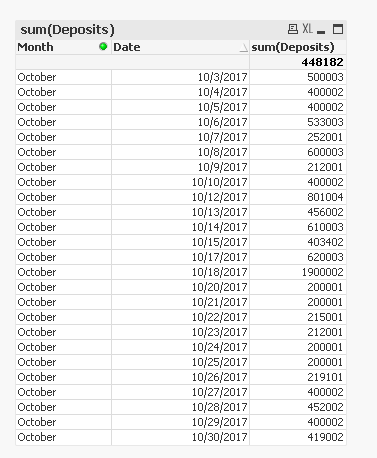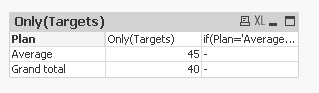# New to QlikView

Discussion board where members can get started with QlikView.

Announcements
Qlik Analytics Tour 2020 Online. Begins August 10th. Register Today
cancel
Showing results for
Did you mean:
HighlightedContributor III

## Set analysis expression to calculate the average

Hello,

I was wondering if I could get some help please.

I am attempting to use an expression to calculate the average total sales for the month in qlikview to put in my summary table.

The raw data displays multiple sales for each day. So I would need to first sum the sales for each business day and then calculate the average for total sales for each day of that month. I have attached an example of the raw data and the desired result which can be achieved in excel. I would like to identify a simple expression to perform this calculation so that I can add it to my pivot summary table in qlikview.

Thanks,

Esther

Tags (2)
1 Solution

Accepted Solutions
HighlightedMVP

## Re: Set analysis expression to calculate the average

May be this

Avg(Aggr(Sum(Deposits), Month, Date))

11 Replies
HighlightedMVP

## Re: Set analysis expression to calculate the average

 I am attempting to use an expression to calculate the average total sales for the month in qlikview to put in my summary table.

Avg({<Month = {\$(=Max(Month))}>} TOTAL [Sum of Deposits])

OR

Avg(TOTAL [Sum of Deposits])

Before develop something, think If placed (The Right information | To the right people | At the Right time | In the Right place | With the Right context)
HighlightedMaster II

## Re: Set analysis expression to calculate the average

Hi,

you can try like below

sum(Deposits) / count(total distinct Month)HighlightedContributor III

## Re: Set analysis expression to calculate the average

Hello,HighlightedMVP

## Re: Set analysis expression to calculate the average

Do you have a month field in your dashboard? May be it is called Month or MONTH?

HighlightedContributor III

## Re: Set analysis expression to calculate the average

Yes I do.

I want to get the result in bold (see screenshot below)in my pivot table but have been unable to get

my expression to work.HighlightedContributor III

## Re: Set analysis expression to calculate the average

The result in bold is the average total for the deposits.

HighlightedMVP

## Re: Set analysis expression to calculate the average

May be this

Avg(Aggr(Sum(Deposits), Month, Date))

HighlightedContributor III

## Re: Set analysis expression to calculate the average

Thank you!HighlightedContributor III

## Re: Set analysis expression to calculate the average

Hello,

The formula works but I am unable to use it in my pivot summary table. Do you know why this could be?

The expression that i am using is this if(Plan='Average',Avg(Aggr(Sum(Deposits), Month, Date)))Thanks